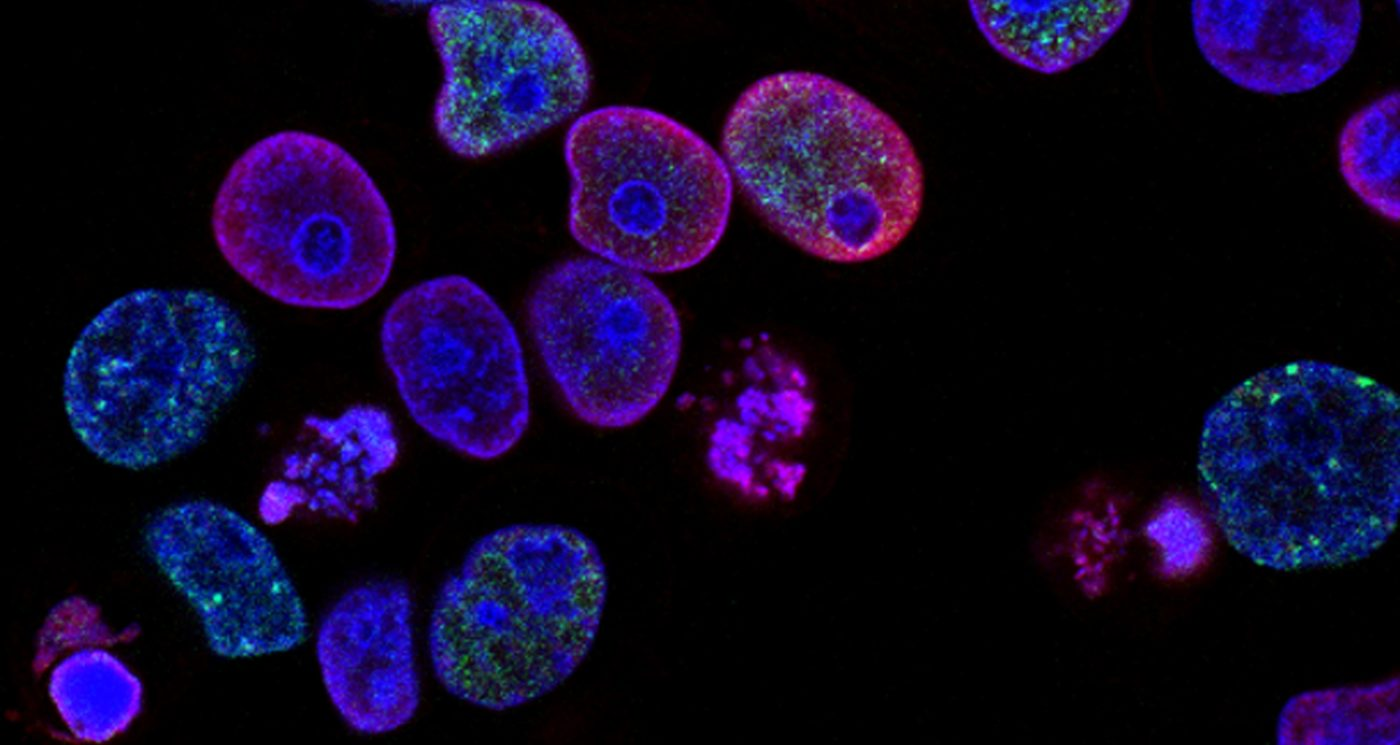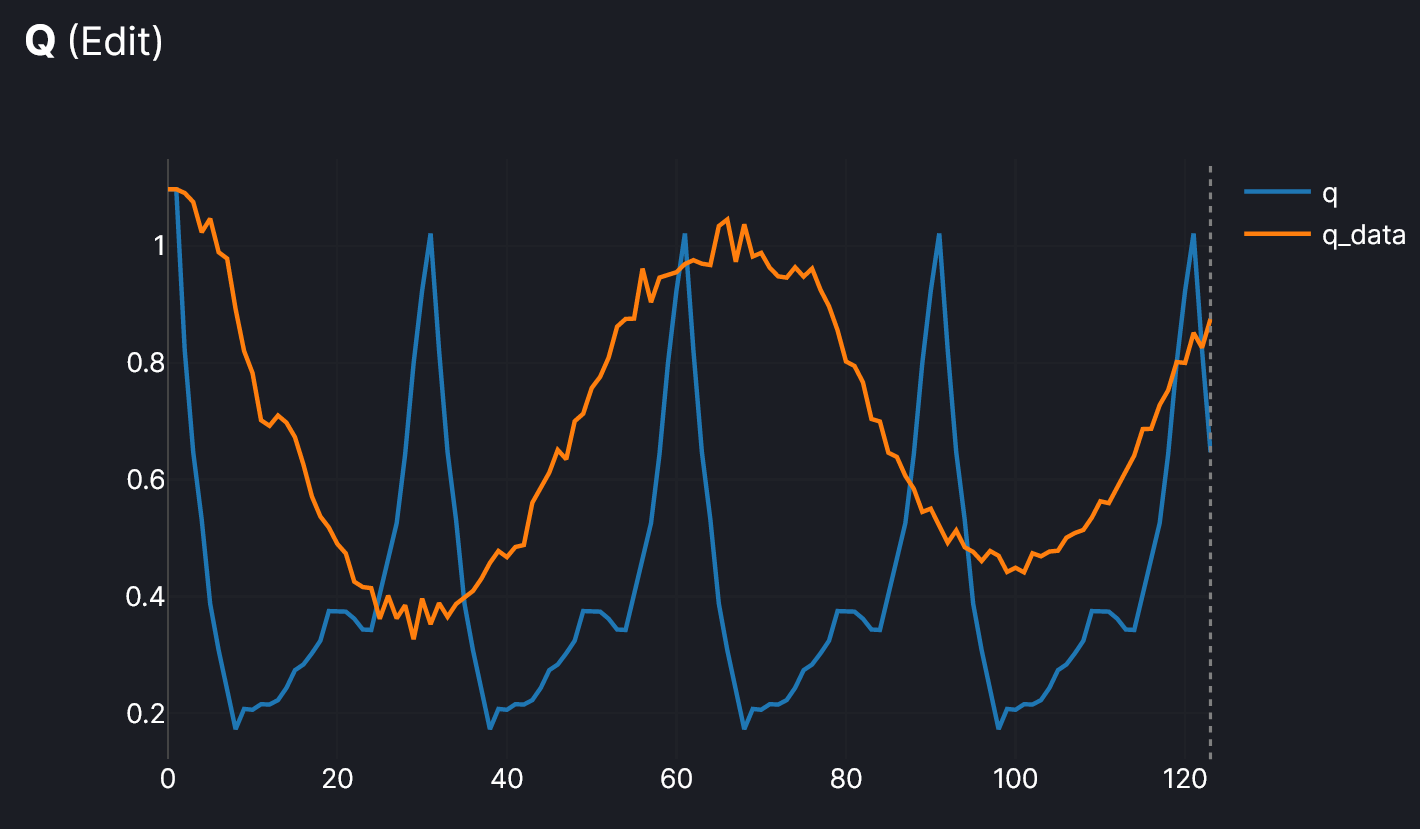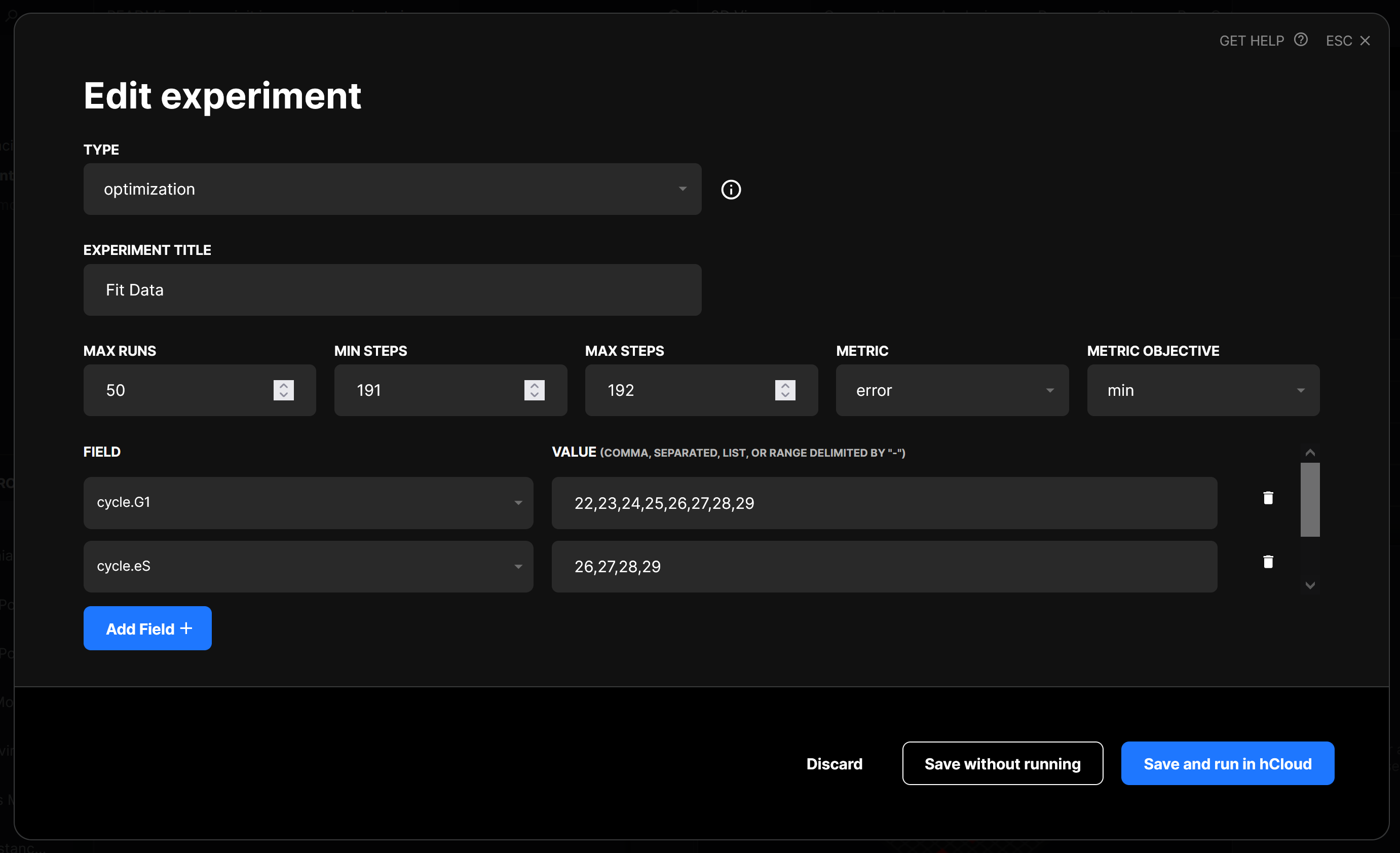# Calibrating Models of Cell ReplicationAgent-based models provide an alternative way of exploring and explaining the world. In this paper, researchers constructed a mathematical model to explain the partial synchronicity that occurs in the replication of cell samples. The growth of a sample of cells is exponential, but discrete.

Because of this, if a sample of cells is completely synchronous (all cells at the same phase of the cycle, replicating at the same time) it will not produce a smooth curve, but rather a discrete step function. To ensure that cell growth in samples is a smooth curve, asynchronous samples are prepared, where cells are randomly distributed across the different phases. However, experimental data shows that most samples behave partially synchronously, not asynchronously. We can attempt to explain this same phenomenon using some simple behaviors in HASH, in conjunction with the optimization engine.

We’ll make one important assumption, and see if it lets us recreate the experimental data. Even though our agents are initially created at random stages in their cell cycle, we’ll assume that when a cell divides, both of them are now synchronous, e.g. at the same stage of their cycle. We’ll mark agents in different colors based on their current cell cycle phase, matching the paper. You can find the model here.

Here’s is how the initial model looked compared to the experimental data (the model is blue and the comparison experimental data is orange):Ration of cells in different stages: Q(t) = R(t) / (Y(t) + G(t))

The general shape looks correct, with exponential growth in the total number of cells, and an oscillating value for Q, although it’s far from close to the data. The growth and period of oscillation are both too fast.

Let’s see if we can use the optimization engine to bring the model much closer to the data. We’ll try to discover the optimal values for the length of each phase in the cell cycle. By inspecting the data, we can see that the period of our cell cycle should be approximately 70 steps long. Let’s choose a range of values for G1, eS, and S/G2/M whose sum is around there.We’ll create a custom agent to compute the error between our model and the experimental data. You can read more about this in the complex metrics section of the HASH docs. At first, let’s try and fit the curves in the second plot, which count the agents based on color.

We can get it looking pretty good, but the 3rd plot, of Q, doesn’t look well fitted. Let’s change the error metric to correspond to the error in Q instead. Here’s the result of that optimization:

It seems like we’ll need to incorporate both of these errors (agent count by colors, and the Q ratio) in order to find the best fit for our model. Since we’ve run some optimizations, we can look at the range of parameters that are producing good results and narrow down our experiment definition, to improve its effectiveness. Instead of a range of ~20 values, we’ll narrow down to a range of between 5-10 for each stage.

Now it looks like we’ve found a set of values that provides the best balance of fit for the 2nd and 3rd plots. The final values for the length of each phase are:

• G1 – 25
• eS – 27
• S/G2/M – 18

Being able to recreate experimental data with agent-based models provides insight into the mechanisms which cause a system to behave in a certain way. Instead of a black-box method, using HASH allows us to answer the question not just of how cell growth happens, but why it happens like that. In this case, we can hypothesize that one explanation for the observed partial synchronicity is that the more cells replicate, the more cells will inherently line up with other cells that share a “parent” cell.